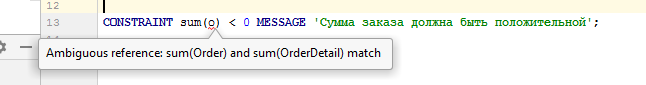Version: 5.x

# How-to: Explicit typing

In certain situations, you may want to use the same name for different system properties or actions.

For example, let's create two properties named `sum`: one of them will calculate the sum of the order line, and the second will calculate the sum of the entire order:

``sum = DATA NUMERIC[10,2] (OrderDetail);sum = GROUP SUM sum(OrderDetail od) BY order(od);``

Accordingly, the first property gets one parameter of the `OrderDetail` class as input while the second gets one parameter of the `Order` class.

But if we create, for example, a constraint with one parameter and then try to refer to the `sum` property without explicitly specifying the class of this parameter, an error will occur:All such references require an explicitly specified class:

``CONSTRAINT sum(Order o) < 0 MESSAGE 'The order amount must be positive';``